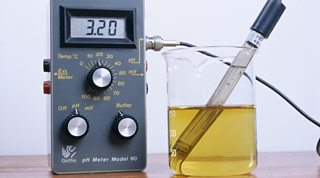Acidic and alkaline solutions

Acids

form in water. Acids are sources of hydrogen , H+. For example, dilute hydrochloric acid forms hydrogen ions:

HCl(aq) → H+(aq) + Cl-(aq)

Alkalis

form solutions in water. These contain hydroxide ions, OH-. For example, dilute sodium hydroxide solution contains hydroxide ions:

NaOH(aq) → Na+(aq) + OH-(aq)

The pH scale

The scale measures the acidity or alkalinity of a solution.

pHMeaning
< 7acidic
= 7neutral
> 7alkaline

Using an indicator

The pH of a solution can be estimated using and a colour chart:

1. dip a clean glass rod into the solution
2. transfer one drop of solution to a piece of universal indicator paper on a white tile
3. wait 30 seconds for the colour to develop, then match the colour to the colour chart
Universal indicator colours

Using a pH meter

The pH of a solution can be measured using a pH meter:

1. wash the pH probe with distilled water
2. put the end of the probe into the solution
3. record the reading on the pH meterThe pH meter may need calibrating first. This is done by adjusting the reading to match the pH of a buffer solution, which is a solution with a known pH.